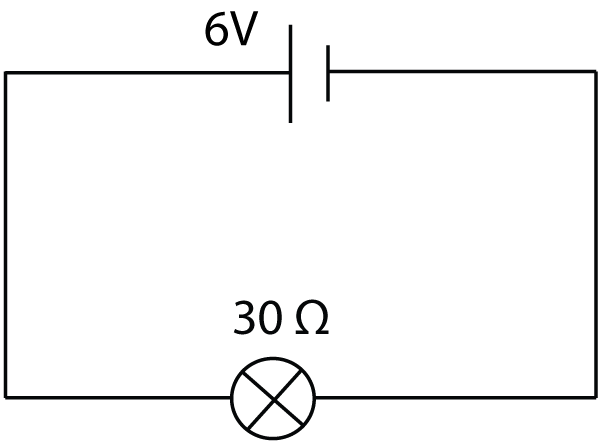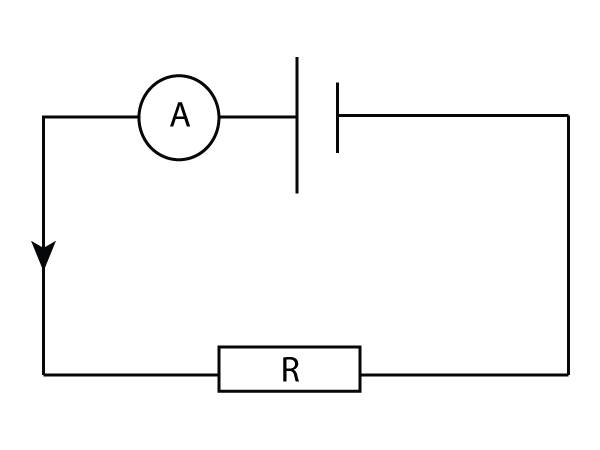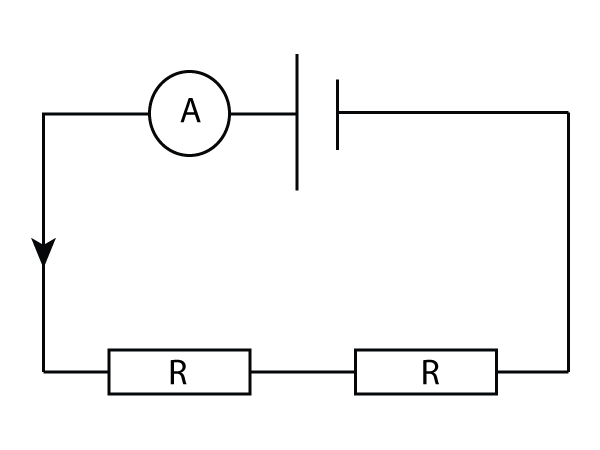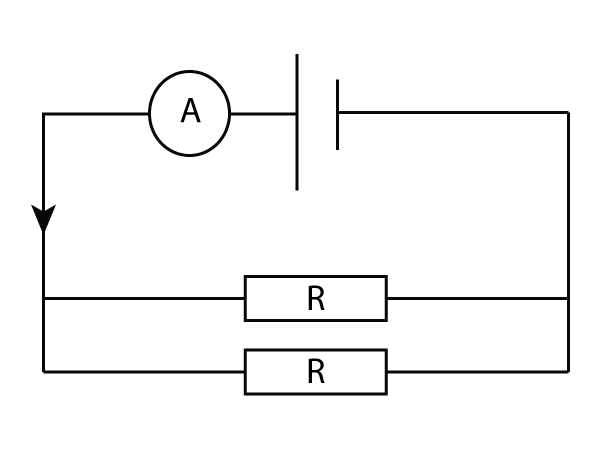10 minutes maximum! Can you do it in 5?

1. Resistance is measured in 'Ω'. This is the symbol for ...

• A. watts.
• B. ohms.
• C. resists.
• D. coulombs.

2. Which of these formulas is used to calculate resistance?

• A. resistance = voltage x (current)2
• B. resistance = voltage ÷ current
• C. resistance = current ÷ voltage
• D. resistance = voltage x current

3. 0.3 kΩ is the same as ...

• A. 3Ω
• B. 30Ω
• C. 300Ω
• D. 3000Ω
4. What is the resistance of this resistor?• A. 0.2Ω
• B. 2Ω
• C. 5Ω
• D. 80Ω
5. What current flows in this circuit?• A. 0.2A
• B. 2A
• C. 5A
• D. 150A

6. What is '2mA' in amps?

• A. 2000A
• B. 200A
• C. 0.02A
• D. 0.002A

7. What is the voltage of this power supply shown in the circuit diagram?• A. 1.5 mV
• B. 6V
• C. 15V
• D. 6000V
8. A steel wire has a resistance. Which of these correctly describes the change to the resistance if the wire is made longer, or thicker? (with a bigger radius).
Longer wire Thicker wire
A
higher resistance lower resistance
B
lower resistance higher resistance
C
lower resistance lower resistance
D
higher resistance higher resistance

Q9 & 10.

In this circuit, a single resistor R is connected to a cell. The total current is given by the ammeter shown.9. If I change the circuit and put 2 of these resistors in series, what happens?• A. The current increases as the total resistance increases.
• B. The current increases as the total resistance decreases.
• C. The current decreases as the total resistance increases.
• D. The current decreases as the total resistance decreases.

10. How does the total current change , compared to a single resistor, if I put 2 of the resistors in parallel?The total current is .